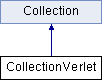ParM  parm A molecular dynamics library
CollectionVerlet Class Reference

#include <collection.hpp>

Inheritance diagram for CollectionVerlet:## Public Member Functions

CollectionVerlet (sptr< Box > box, sptr< AtomGroup > atoms, const flt dt, vector< sptr< Interaction > > interactions=vector< sptr< Interaction > >(), vector< sptr< StateTracker > > trackers=vector< sptr< StateTracker > >(), vector< sptr< Constraint > > constraints=vector< sptr< Constraint > >())

void timestep ()
Take one step forward in time. More...

void set_dt (flt newdt)Public Member Functions inherited from Collection
Collection (sptr< Box > box, sptr< AtomGroup > atoms, vector< sptr< Interaction > > interactions=vector< sptr< Interaction > >(), vector< sptr< StateTracker > > trackers=vector< sptr< StateTracker > >(), vector< sptr< Constraint > > constraints=vector< sptr< Constraint > >(), bool should_initialize=true)

virtual void initialize ()

virtual void set_forces (bool constraints_and_a=true)
Set forces. More...

flt degrees_of_freedom ()
Total degrees of freedom. More...

flt potential_energy ()

flt energy ()
Total energy, including both potential and kinetic_energy. More...

virtual flt temp (bool minuscomv=true)

virtual flt kinetic_energy ()

virtual flt virial ()

virtual flt pressure ()
Returns (1/d V) Σ ri dot fi where d is number of dimensions note that Interaction->pressure just returns Σ ri dot fi. More...

sptr< Boxget_box ()

Vec com ()

Vec com_velocity ()

Vec angular_momentum (const Vec &loc)
Shortcut to AtomGroup method of the same name. More...

Vec angular_momentum ()
Shortcut to AtomGroup method of the same name. More...

virtual ~Collection ()

void reset_com_velocity ()
Shortcut to AtomGroup method of the same name. More...

void reset_L ()
Shortcut to AtomGroup method of the same name. More...

void scale_velocities (flt scaleby)
Scale all velocities by a factor. More...

void scale_velocities_to_temp (flt T, bool minuscomv=true)
Scale all velocities to get to a specific temperature. More...

void scale_velocities_to_energy (flt E)
Scale all velocities to get to a specific total energy. More...

void add_interaction (sptr< Interaction > inter)

void add_tracker (sptr< StateTracker > track)

void add_constraint (sptr< Constraint > c)

void add (sptr< Interaction > a)

void add (sptr< StateTracker > a)

void add (sptr< Constraint > a)

vector< sptr< Interaction > > get_interactions ()

## Protected Attributes

flt dtProtected Attributes inherited from Collection
sptr< Boxbox

sptr< AtomGroupatoms

vector< sptr< Interaction > > interactions

vector< sptr< StateTracker > > trackers

vector< sptr< Constraint > > constraintsProtected Member Functions inherited from Collection
void update_trackers ()
To be called immediately after setting particle positions and velocities; lets StateTracker instances stay updated automatically. More...

void update_constraint_positions ()
To be called approximately after forces have been set. More...

void update_constraint_velocities ()

void update_constraint_forces ()

virtual flt set_forces_get_pressure (bool constraints_and_a=true)

Examples:
LJatoms.cpp.

## Constructor & Destructor Documentation

 CollectionVerlet::CollectionVerlet ( sptr< Box > box, sptr< AtomGroup > atoms, const flt dt, vector< sptr< Interaction > > interactions = vector >(), vector< sptr< StateTracker > > trackers = vector >(), vector< sptr< Constraint > > constraints = vector >() )
inline

## Member Function Documentation

 void CollectionVerlet::set_dt ( flt newdt )
inline
 void CollectionVerlet::timestep ( )
virtual

Take one step forward in time.

Implements Collection.

Examples:
LJatoms.cpp.

## Member Data Documentation

 flt CollectionVerlet::dt
protected

The documentation for this class was generated from the following files: